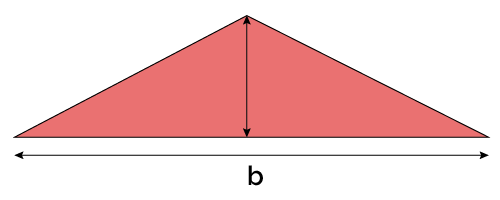# Obtuse Triangle Calculator

Created by Łucja Zaborowska, MD, PhD candidate
Reviewed by Gabriela Diaz
Last updated: Jun 10, 2022

Welcome to the one and only obtuse triangle calculator - wonder no more; this simple tool will not only calculate the area of your triangle but also determine whether it's really obtuse or not!

• How to calculate the area of an obtuse triangle; 🔺
• How to find out whether your triangle is obtuse; and
• How to use the obtuse triangle calculator.

## What is an obtuse triangle?

"Obtuse" describes a triangle that comprises:

• 1x angle that measures over 90 degrees (>90°), called an obtuse angle; and
• 2x angles that measure less than 90 degrees (<90°), called the acute angles.

The obtuse triangle is one of two types of oblique triangles - the other one is acute.

## How to determine whether the triangle is obtuse?

Angles are all you need to determine whether the triangle is obtuse!

1. Take a look at your known triangle angles - if one of them is greater than 90 degrees, your triangle is obtuse. ✅
2. If you know only 2 angles of your triangle, use the equation below:

Angle(α) = 180 - Angle(β) - Angle(γ)

## How to calculate the area of an obtuse triangle?

You can calculate the area of an obtuse triangle using every typical equation for a triangle area.

Let's enumerate a few examples:

• Area = 0.5 × Base × Height
• Area = 0.25 × √( (a + b + c) × (-a + b + c) × (a - b + c) × (a + b - c) )
• Area = 0.5 * a × b × sin(γ)

Where:

• a, b, and c are the length of the obtuse triangle's sides.

## How to use the triangle type calculator?

It's probably the easiest thing on earth! 🌍

Enter one, two, or three angles of your triangle - our obtuse triangle sides calculator will show you the answer straight away!

Aside from identifying whether or not your triangle is obtuse, you may also use our tool to calculate its area! Go ahead and choose from multiple options available.

## The full list of triangle area calculators:

The obtuse triangle calculator is a part of a bigger series of tools - discover them all! 🛠️

## FAQ

### What triangle type has an angle greater than 90 degrees?

An obtuse triangle has exactly one angle greater than 90° - called the obtuse angle.

Two other angles of the obtuse triangle are acute - they measure less than 90°.

Łucja Zaborowska, MD, PhD candidate
Is my triangle obtuse?
Angle α
deg
Angle β
deg
Angle γ
deg
Obtuse triangle area
Given
base and heightBase
in
Height
in
Area
in²
People also viewed…

### Acute triangle

The acute triangle calculator helps you decide if a given triangle belongs or not to the class of acute triangles.

### Car heat

The hot car calculator shows you how fast the interior of a car heats up during a summers day.

### Circle length

Calculate the circle length with this fantastic tool, and know its circumference, radius, or diameter.

### Sunbathing

Do you always remember to put on sunscreen before going outside? Are you sure that you use enough? The Sunbathing Calculator ☀ will tell you when's the time to go back under an umbrella not to suffer from a sunburn!